﻿ 因素空间理论——机制主义人工智能理论的数学基础
«上一篇文章快速检索 高级检索

 智能系统学报2018, Vol. 13Issue (1): 37-54  DOI: 10.11992/tis.2017110340

### 引用本文WANG Peizhuang. Factor space-mathematical basis of mechanism based artificial intelligence theory[J]. CAAI Transactions on Intelligent Systems, 2018, 13(1): 37-54. DOI: 10.11992/tis.201711034.### 文章历史

Factor space-mathematical basis of mechanism based artificial intelligence theory
WANG PeizhuangCollege of Intelligence Engineering and Mathematics, Liaoning Technical University, Fuxin 123000, China
Abstract: Based on using the intelligent growth mechanism, the mechanism-based artificial intelligence theory organically unifies the structure, function, and behaviorism of three genres to form a trinity of consciousness, emotion, and reason. Factor space is the mathematical basis of mechanism-based artificial intelligence theory, which promotes mathematical branches such as formal concept analysis, rough sets, and fuzzy sets, and provides a universal coordinate framework for the description and cognition of things. Data can be represented as visual sampling points in the space and then be cultivated to form the population distribution of the background relation. Based on their relationship, concept generation and causality analysis can be performed automatically, and all rational thinking processes, such as prediction, identification, control, evaluation and decision making, can be performed by factorial algorithms. In this article, we focus on ways to describe formal information (i.e., grammatical information), predict utility information (i.e., pragmatic information) from formal information, and correlate them to generate abstract semantic information, which is helpful for mathematically describing the first established law of information transformation in mechanism-based artificial intelligence theory. We also use factor space theory in chess Tic-Tac-Toe to demonstrate how to dock the target and chess factors, which may provide a clue for how to mathematically describe the second law of information transformation. We also provide a brief history to help readers gain a more comprehensive understanding of the factor space theory.
Key words: mechanism-based artificial intelligence theory    factor space theory    formal concept analysis    rough sets    fuzzy sets    falling shadow theory    background relation    datamining

1 因素空间的基本框架

1.1 因素与因素空间的数学定义

 $X\left( F \right) = X\left( {{f_{\left( 1 \right)}}} \right) \times X\left( {{f_{\left( 2 \right)}}} \right) \times \cdots \times X\left( {{f_{\left( k \right)}}} \right)$ (1)

 $F \cup G = {\rm{Sup}}\left\{ {F,\;G} \right\},\;F \cap G = {\rm{Inf}}\left\{ {F,\;G} \right\}$ (2)

$F^* = {f_1} \cup {f_2} \cup \cdots \cup {f_n}$ 是在所定义的系统中最大的因素，叫作全因素。由此还可以定义余运算c ${F^c}$ 的性状空间是那些不被F所包含的元性状空间的乘积。不难证明 $P\left( {F^*} \right) = (P(F^*), \cup , \cap {,^c})$ 形成一个因素布尔代数。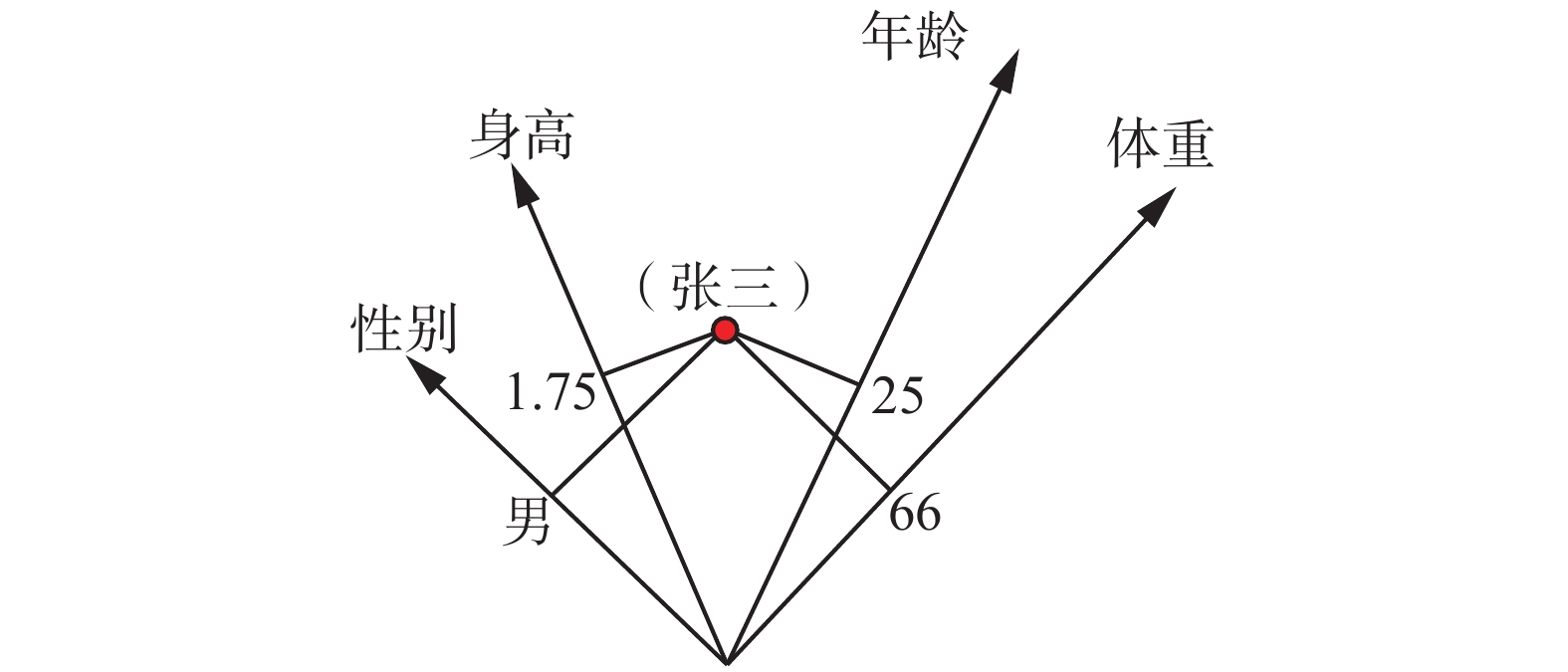Download: 图 1 张三被映射为因素空间中的一个点 Fig. 1 Mapping Zhang San mapped to a point in the factor space
1.2 背景关系与背景分布

 $\left[ {\mathit{\boldsymbol{a}}} \right] = {F^{ - 1}}\left( {\mathit{\boldsymbol{a}}} \right) = \left\{ {u \in U\left| {F\left( u \right) = {\mathit{\boldsymbol{a}}}} \right.} \right\}$ (3)

$\left[ {\mathit{\boldsymbol{a}}} \right]$ 可能是空集 $\phi$ ，若 $\left[ {\mathit{\boldsymbol{a}}} \right] \ne \phi$ ，则称a是一个实性状颗粒，否则称a是一个虚组态。全体实性状的集合记为

 $\begin{array}{c}R = F\left( U \right) = \left\{ {{\mathit{\boldsymbol{a}}} = \left( {{a_1},{a_2}, \cdots ,{a_n}} \right) \in X|\exists u \in U;} \right.\\\left. {{f_1}\left( u \right) = {a_1},{a_2}, \cdots ,{f_n}\left( u \right) = {a_n}} \right\}\end{array}$ (4)

 $R = \left\{ {a \in X|p\left( a \right) > 0} \right\}$ (5)

 $R = \cap \left\{ {B \in {\mathit{\boldsymbol{B}}}\left| {{{\smallint}}_B {P\left( x \right)} {\rm{d}}{\mathit{\boldsymbol{x}}} = 1} \right.} \right\}$ (6)
1.3 因素数据库与背景基

$S$ 是相样本，始终有 $S \subseteq R$ ；所有同表头相样本的并就是背景关系：

 $R = \cup \left\{ {S|S{\text{是相样本}}} \right\}$ (7)

 $(o - d,a - d) = (1.7, - 1)(0, - 3) = 3 > 0$
 $(o - d,b - d) = (1.7, - 1)\left( {2,1} \right) = 2.4 > 0$

$(o - d,c - d) = (1.7, - 1)(3, - 1) = 6.1 > 0$

 $(o - e,a - e) = \left( {0.7,{\rm{ }}1} \right)( - 1, - 1) = - 1.7 < 0$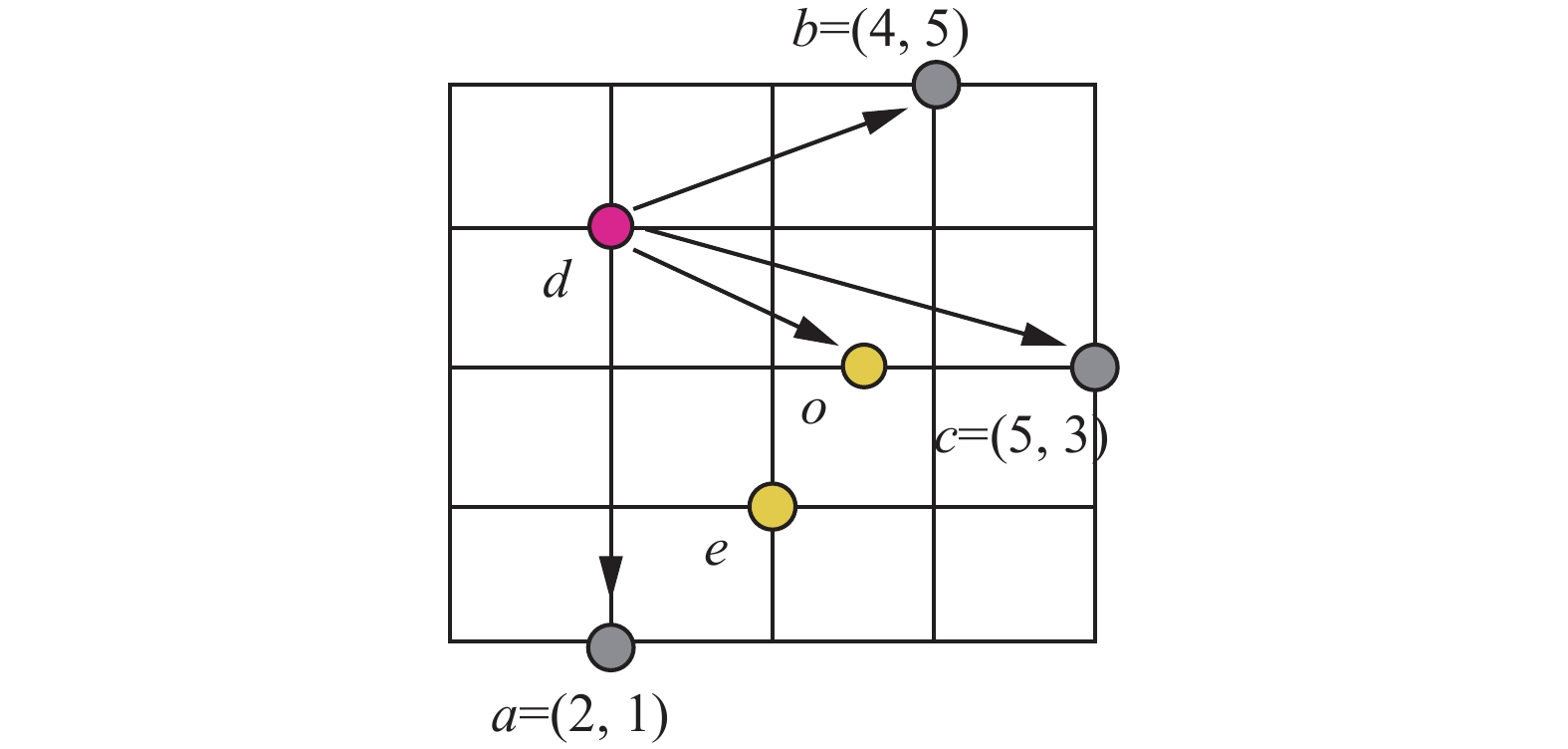Download: 图 2 背景基内点的夹角判别法 Fig. 2 The angle criterion for inner points of background base

1.4 因素藤、因素神经网络与智联网

2 形式概念的生成与基本概念半格的提取

1982是不平凡的一年，在这一年中同时出现了3个数学分支，公开宣称以知识和智能作为自己的数学研究对象。在这3个分支中，首先要介绍的是德国数学家R. Wille所提出的形式概念分析(normal concept analysis)。在他以前，数学家们认为，任何概念的外延都是集合，而任何集合都是概念的外延。Wille明确地对后一句话说不。他提出了内涵和外延的对合性准则，认为只有满足对合性，才能把一个集合视为一个概念的外延。给定对象集 $O$ 和属性集 $A$ ，从它们之中分别取出一组对象 $E$ 和一组属性 $I$ ，记 $f\left( E \right) = \left\{ {a \in A|\forall o \in E;o{\text{具有属性}}a} \right\}$ ,它是 $E$ 中对象共有属性所形成的集。记 $g\left( I \right) = \left\{ {o \in O|} \right.$ $\left. {\forall a \in I;o{\text{具有属性}}a} \right\}$ ，它是 $I$ 中属性共同依附的对象所形成的集。如果 $f\left( E \right) = I$ 且记 $g\left( I \right) = E$ ，则称配对 $a = \left( {I, E} \right)$ 满足对合性，并称 $a$ 是一个以 $I$ 为内涵和以 $E$ 为外延的概念。表 1 科教电影“生物与水”的背景 Tab.1 The background of science and education film "biology and water"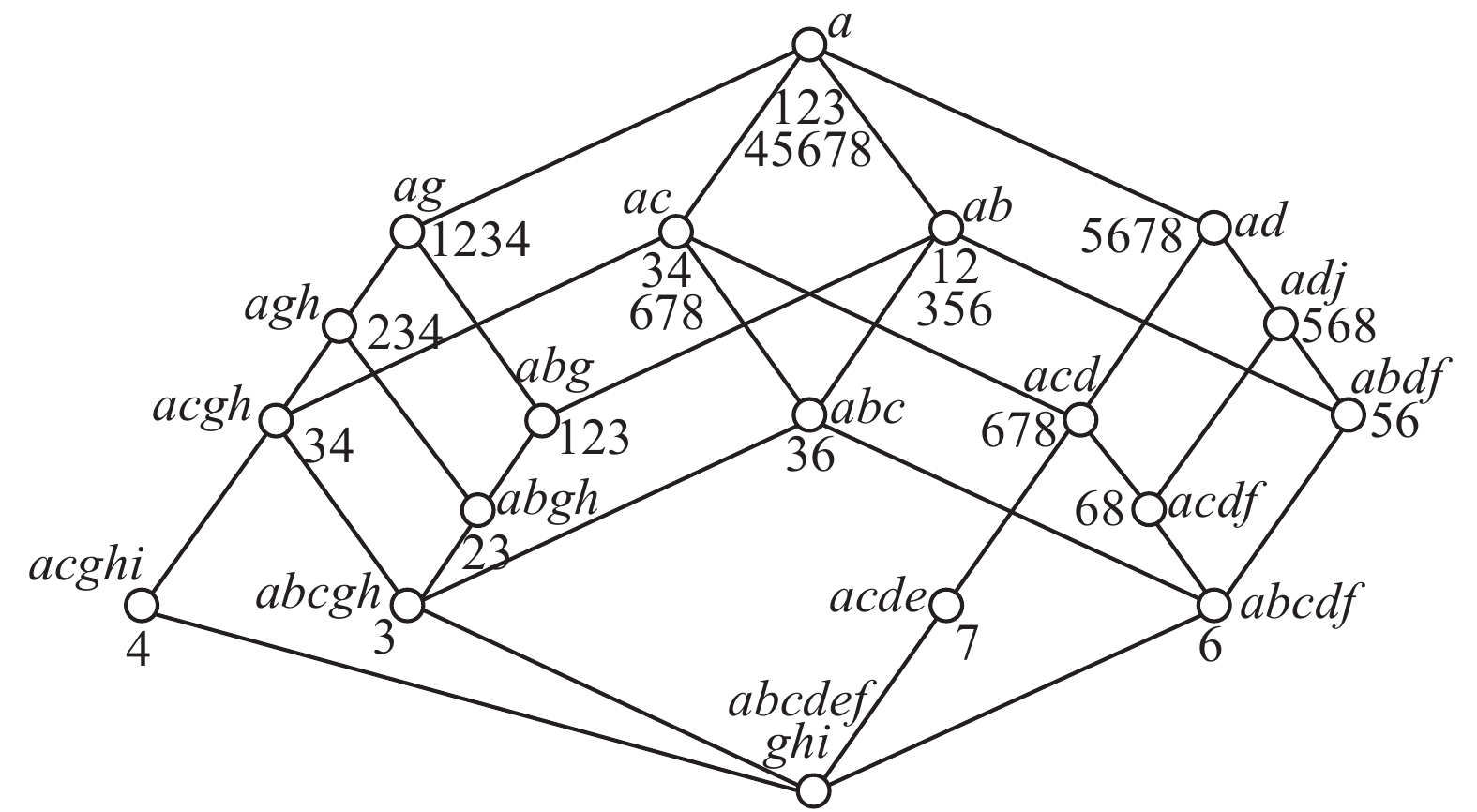Download: 图 3 “生物与水”的‘概念格’图 Fig. 3 The concept lattice of "biology and water"

Wille的工作缺陷在于：1)他的形式背景表以属性来分列，制造了列表困难，由此导致他的算法复杂。为了寻找对合的概念，从每一行或列开始搜索，再每两行或两列 $\cdots \cdots$ 这样的搜索方式本身就是指数爆炸的。他一整本书就是为了避免指数爆炸而设立各种算法，但仍然无法摆脱N-hard陷阱。2)Wille所说的概念，都是属性的析取，只含“且”字而不含“或”字，这不是一般概念而是基本概念。对合性只对基本概念成立，带或字的概念是无法对合的。基本概念只能形成半格，所以，他说的概念格应该改为基本概念半格。

 ${d_f} = 1 - [n\left( 1 \right)(n\left( 1 \right) - 1) + \cdots + n\left( K \right)(n\left( K \right) - 1)]/m(m - 1)$ (8)

${\mathit{\boldsymbol{a}}}$ $\left[ {\mathit{\boldsymbol{a}}} \right]$ 都是原子，由于 $F$ 是从 $H\left( {U,F} \right)$ $R$ 的同构映射，它们一定满足Wille的对合性。

1) 给定U，计算每个因素对U中对象的分辨度；

2) 选对U分辨度最大的因素f来实现fU的分类: 置换对象足码即（行足码）使同类对象连接在一起；

3) 用所分出的子类U′取代U, 重复步骤1）和2），直到所有的子类都变成粒子为止，总结出粒子半格。表 2 成员状况因素表 Tab.2 Member status factors

$X$ (性别)={男，女}， $X$ (身高)={高，中，低}，X(体重)={重，常，轻}，现在的问题是要生成概念布尔代数。

$X = X\left( {{f_1}} \right) \times X\left( {{f_2}} \right) \times X\left( {{f_3}} \right) =$ {男高重，男高常，男高轻，男中重，男中常，男中轻，男低重，男低常，男低轻，女高重，女高常，女高轻，女中重，女中常，女中轻，女低重，女低常，女低轻}

$X$ 被分成18个格子，去掉其中的虚组态，得到 $U$ 上3个因素所形成的背景关系：

$R =$ {男高重，男高常，男中常，男中轻，女中重，女中常，女低常，女低轻}

[男高重]={1, 2, 3}, [男高常]={4, 5}, [男中常]={6, 7, 8}, [男中轻]={9, 10}, [女中重]={11, 12}, [女中常]={13, 14, 15}, [女低常]={16}, [女低轻]={17, 18, 19, 20}

${\alpha _1}$ =(男高重, {1, 2, 3}), ${a_2}$ =(男高常, {4, 5}), ${\alpha _3}$ =(男中常, {6, 7, 8}), ${\alpha _4}$ =(男中轻, {9, 10}), ${\alpha _5}$ =(女中重, {11, 12}), ${\alpha _6}$ =(女中常, {13, 14, 15}), ${\alpha _7}$ =(女低常, {16}), ${\alpha _8}$ =(女低轻, {17, 18, 19, 20})

${\alpha _1} \vee {\alpha _5}$ =(男高重,{1, 2, 3}) $\vee$ (女中重, {11, 12})=(男高重或女中重, {1, 2, 3, 11, 12})

1) 计算分辨度： $m = 14$

 $\begin{array}{c} {f_1} = {\text{性别}},\;n\left( 1 \right) = 10,\;n\left( 2 \right) = 10,\\{c_f}_1 = 1 - (10 \times 9 + 10 \times 9)/\left( {20 \times 19} \right) = \\ 1 - 180/380 = 1 - 9/19 = 10/19 \end{array}$
 $\begin{array}{c} {f_2} = {\text{身高}},\;n\left( 1 \right) = 5,\;n\left( 2 \right) = 10,\;n\left( 3 \right) = 5,\\{c_f}_2 = 1 - (5 \times 4 + 10 \times 9 + 5 \times 4)/\left( {20 \times 19} \right) = \\ 1 - 130/380 = 25/38 \end{array}$
 $\begin{array}{c} {f_3} = {\text{体重}},\;n\left( 1 \right) = 5,\;n\left( 2 \right) = 9,\;n\left( 3 \right) = 6,\\{c_f}_3 = 1 - (5 \times 4 + 9 \times 8 + 6 \times 5)/\left( {20 \times 19} \right) = \\ 1 - 112/380 = 268/380 \end{array}$

${c_f}_3 > {c_f}_2 > {c_f}_1$

2) 按因 ${f_3}$ (体重)进行足码置换，使对象按重、常、轻分类排列(见表3)。表 3 表2的变换 Tab.3 Transformation of table 2

 $\begin{array}{*{20}{c}}{U = {C_1}\left\{ {1,2,3,11,12} \right\} + {C_2}\left\{ {4,5,6,7,8,13,14,15,16} \right\}} + \\{{C_3}\left\{ {9,10,17,18,19,20} \right\}}\end{array}$

3) 再就因素 ${f_2}$ (身高)逐类考察，看看该类对象是否按高、中、低分相排列。在 ${C_1}$ 中，需要对对象 $k$ $c$ 的位置进行置换，得到表4表 4 表3的转换 Tab.4 Transformation of table 3

 ${C_2} = {C_{21}}\left\{ {4,5} \right\} + {C_{22}}\left\{ {6,7,8,13,14,15} \right\} + {C_{23}}\left\{ {16} \right\}$

3类分别与内涵常高、常中、常低对合，得到3个基本概念 ${\beta _{21}} = ({\text{常高}},{C_{21}})$ ${\beta _{22}} = ({\text{常中}},{C_{22}})$ ${\beta _{23}} =$ $({\text{常低}},{C_{23}})$

 ${C_3} = {C_{31}}\{ 9,10\} + {C_{32}}\{ 17,18,19,20\}$

4) 再就因素 ${f_3}$ (性别)逐类考察，看看该类对象是否男女分相排列. 发现性别在所有类中的排列都无需置换，类似可将 ${C_{22}} = {C_{221}}\left\{ {6,7} \right\} + {\rm{ }}{C_{221}}\left\{ {8,13,14,15} \right\}$ 得到两个基本概念 ${\beta _{221}} = ({\text{常中男}},{C_{221}}),{\beta _{222}} =$ $({\text{常中女}},$ C222)。所有类都无法再划分。

5) 画出基本概念半格图(见图4)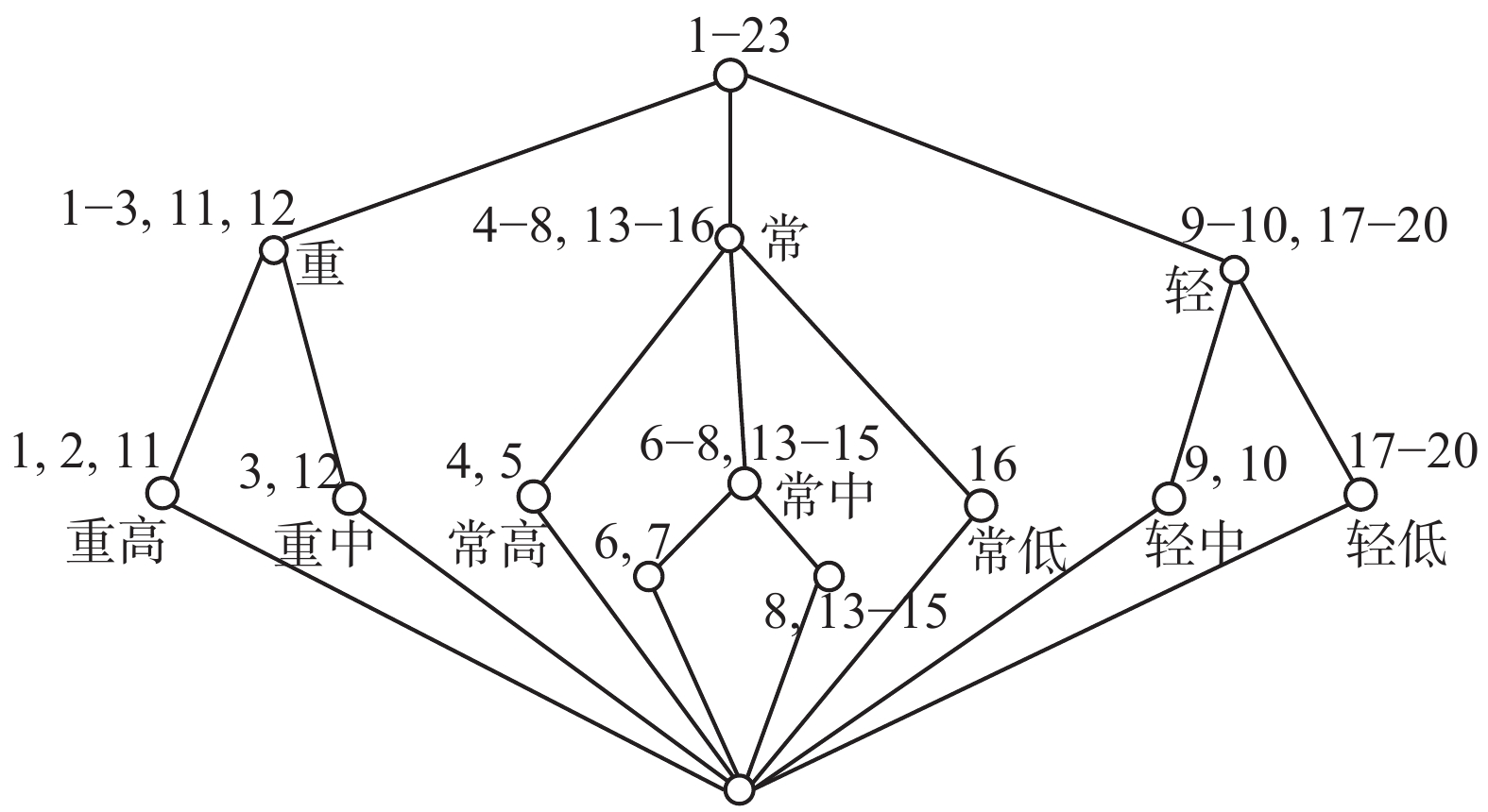Download: 图 4 成员状况粒子半格 Fig. 4 Member state particle semi-lattice表 5 将形式背景表按因素并列 Tab.5 Juxtaposition of formal background tables by factors表 6 将形式背景表分解成两个因素性状表 Tab.6 Form background table is decomposed into two factor traits表 7 两个因素性状表的化简 Tab.7 Simplification of the table of two factors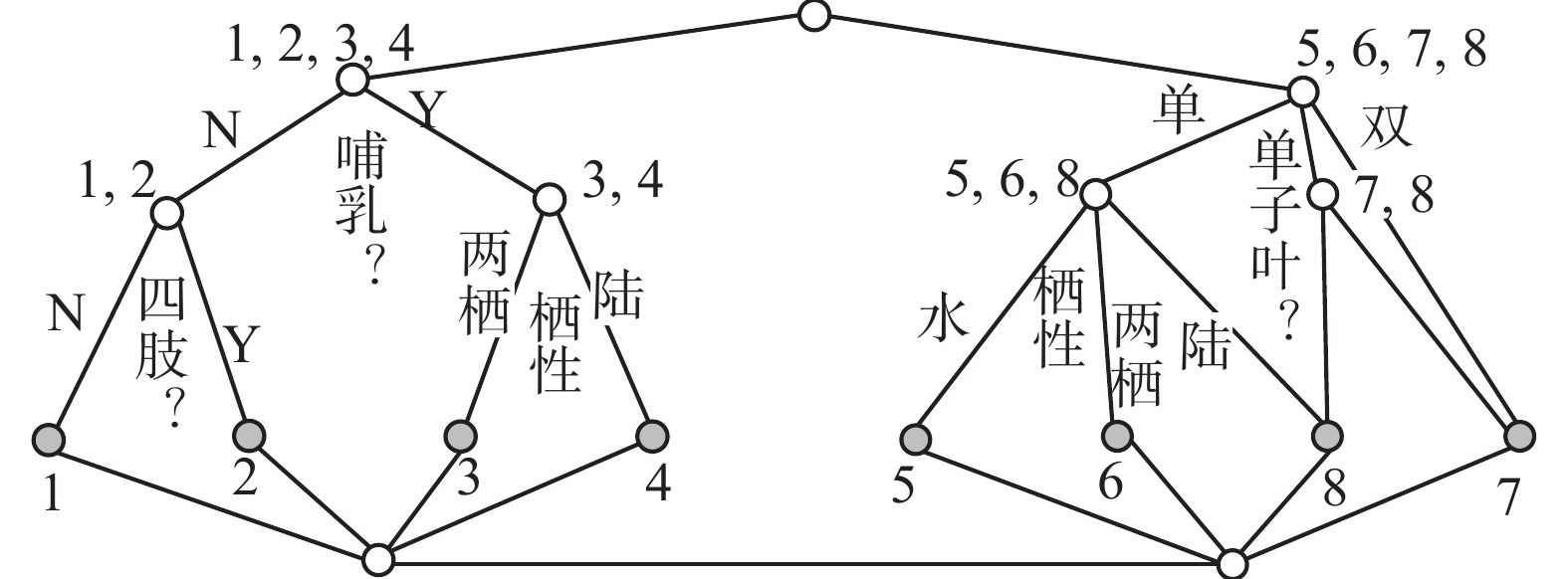Download: 图 5 因素空间对生物与水的形式概念分析图 Fig. 5 Formal concept analysis for biological and water based on factors space

Wille不知道机制主义的人工智能理论，对形式(语法)信息、效用信息和语义全信息不加区分，他所举的例子都是语法信息。所以，本节可以视为形式信息的提取。至于如何用因素空间将形式信息与效用信息向语义信息，提升，是我们在下一节要做的事情。

3 因素之间的关联，语义信息的提升

 $R = X\left( {{f_1}} \right) \times X\left( {{f_2}} \right) \times \cdots \times X\left( {{f_n}} \right)$ (9)

$n = 2$ ，采用1.2节中约定的简单记号。若 $R \ne X\left( {{x}} \right) \times X\left( {{y}} \right)$ ，则因素 ${{x}}$ ${{y}}$ 之间互有关联。对任意 $x \in X$ ，记 $R\left( x \right) = \{ y \in Y|\left( {x,y} \right) \in R\}$ ，叫作 $R$ $x$ 点的截集，它是 $Y$ 的子集。

${R_X} = \{ x|\exists y \in Y;{\rm{ }}\left( {x,y} \right) \in R\}$ ${R_Y} = \{ y|\exists x \in X;$ ${\rm{ }}\left( {x,y} \right) \in R\}$ 分别叫作 $R$ $X$ $Y$ 中的投影。 ${\left( {{R_X}} \right)^c}$ 叫作 ${{x}}$ ${{y}}$ 的盲区， ${\left( {{R_Y}} \right)^c}$ 叫作 ${{y}}$ ${{x}}$ 的盲区。排除盲区可以提高搜索效率。

$R$ 的领域越小，关联性越强。当小到只有一个性状颗粒 $(a,b)$ 的时候，则输入 $x$ 的任意前提 $A$ ，关联到 ${\mathit{\boldsymbol{y}}}$ 只有两种可能：若 $A$ 包含 $a$ ，则 $y = b$ ，否则 $y \ne b$ 。当 $b$ 是关键性状时， $R$ 可以用作由 ${\mathit{\boldsymbol{x}}}$ ${\mathit{\boldsymbol{y}}}$ 的精准预报器。

 $\left( {E \times Y} \right) \cap R \subseteq \left( {X \times {\rm E}'} \right) \cap R$ (10)

 $(E \times Y){ \subseteq _R}(X \times E')$ (11)Download: 图 6 背景关系决定因果 Fig. 6 Determinants of background relations

1) 计算各个因素 ${f_j}$ 对结果 $g$ 的决定度；

2)选决定度最大的因素对 $U$ 分类，若出现能钻入结果类的类别，就写出相应的因果句；逐次细化分类，直到所分类全部钻入结果类或不能再分为止。所有因果句的全体称为因果规则集。表 8 因果关联分析表 Tab.8 Causal association analysis table表 9表8的删除而得的表 Tab.9 A table obtained by the deletion of table 8

4 用因素空间下九宫棋

1) 确立目标，选定目标因素

2) 注意对象，确定对象因素

3) 目标因素与对象因素的对接表 10 一字棋的因果关联分析表 Tab.10 A causal link analysis table for Chinese characters

4) 精细化与棋谱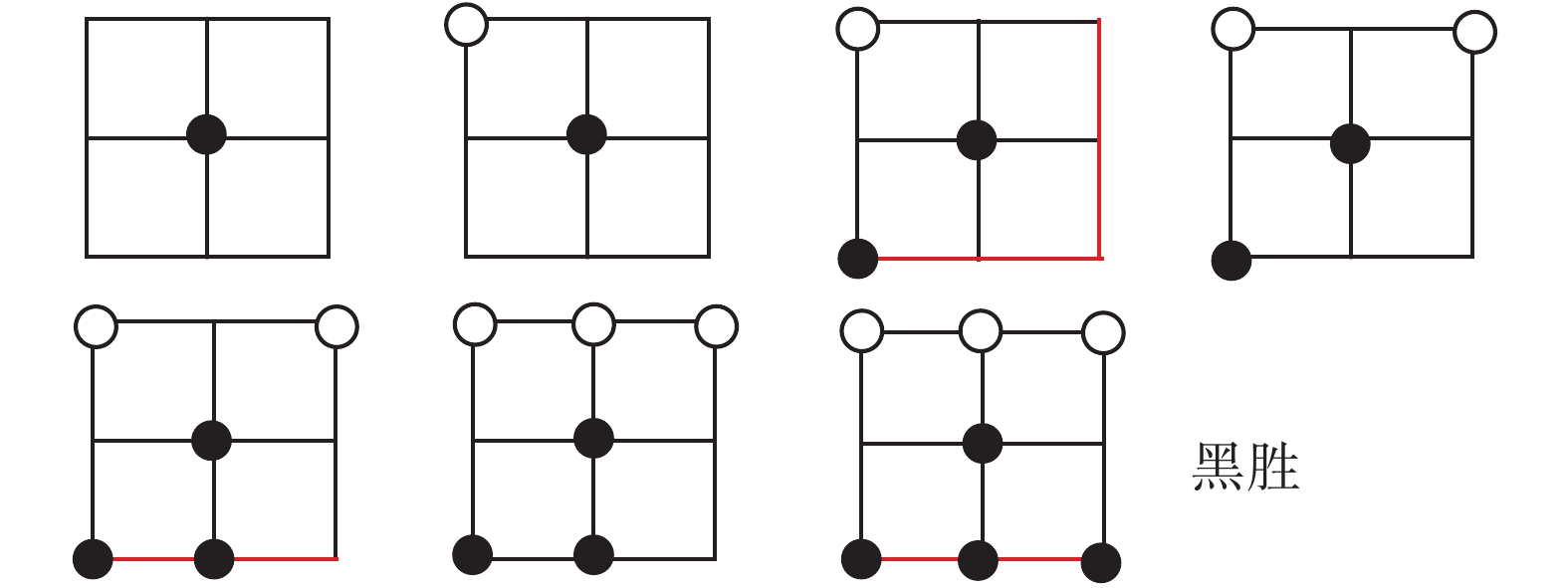Download: 图 7 棋谱1的图示 Fig. 7 Chess 1 Icon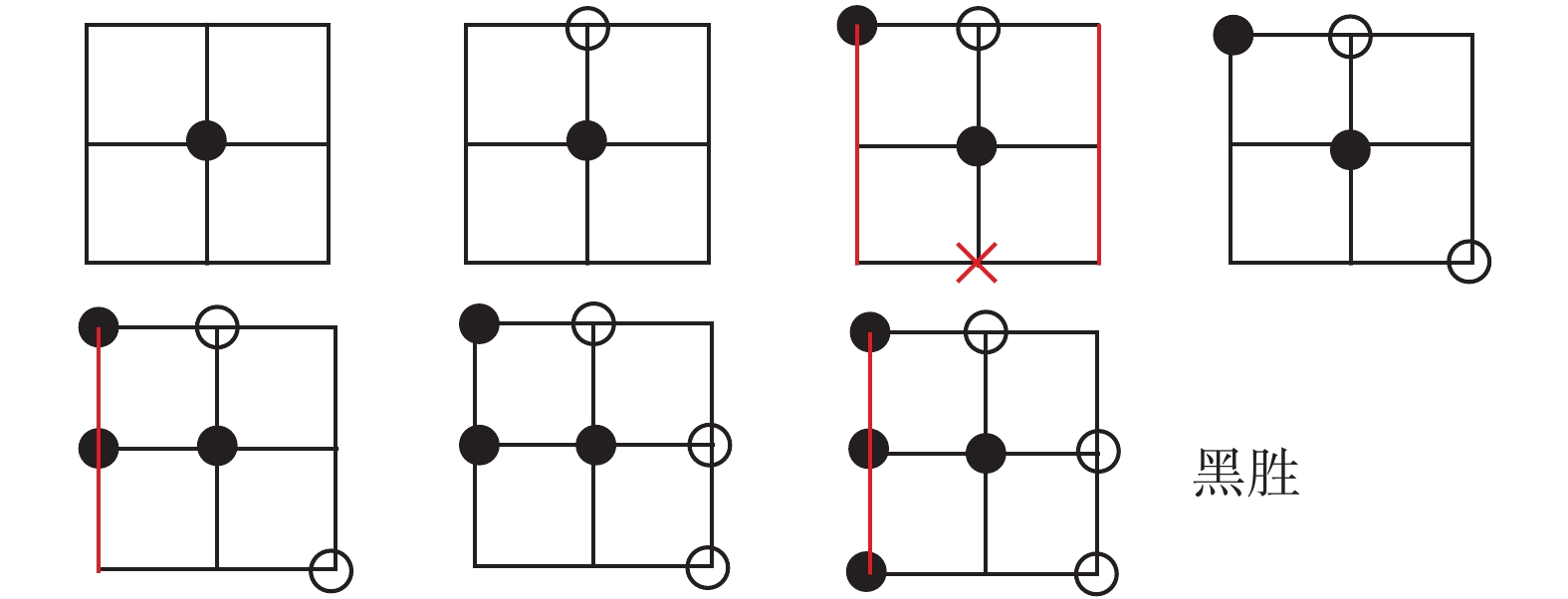Download: 图 8 棋谱2的图示 Fig. 8 Chess 2 Icon

5 因素空间的历史与未来

 $\xi = f\left( x \right) + {f^ \wedge}\left( x \right) + \delta$ (12)

1965年，Zadeh教授提出了模糊集合论，这是人工智能在数学上取得突破的重要历史进展。他把模糊集定义成从论域 $U$ 到[0, 1]上的一个映射 ${\mu _A}:U \to \left[ {0,1} \right]$ ${\mu _A}\left( u \right)$ 表示 $u$ $A$ 的隶属程度；至于论域 $U$ 是什么，则很少有人问津。然而，论域是刻画模糊信息极为重要的一环，笔者提出因素空间的理论来刻画论域，以实现模糊性与清晰性之间的相互转换。例如，年轻是一个模糊概念，要看一个人是否年轻，单就年龄这个因素打分(确定隶属度)相当困难。若加上因素面貌，打起分来就容易一些；若再加上精力、体魄、反应、朝气等因素，打分就更容易了。问题的关键是因素的增加会降低认识的模糊性。

2012年以后，笔者将因素空间的研究重点转向数据智能化的数学理论，与形式概念分析及粗糙集结缘。现有的关系数据库所面临的大数据的挑战是因素空间所要迎头解决的首要问题。因素空间对形式信息的处理对象囊括了非结构化数据。以因素空间为基础的机制主义人工智能是数据智能化的灵魂，它不俯首听命于大数据的摆布，而是要设计、制造、运用和培育数据，用数据来营造信息生态和知识生态，建立信息、知识与神经结构的同构体。它要改变当今世界人工智能自下而上的手工式格局，代之以自上而下与自下而上相结合的发展理念。有许多文章可以参考[25-50]，尽管作者们在写作的时候还没有形成自己思维的大局。

6 结束语

  钟义信. 高等人工智能原理: 观念•方法•模型•理论[M]. 北京: 科学出版社, 2014. (0)  钟义信. 信息科学与技术导论[M]. 3版. 北京: 北京邮电大学出版社, 2015. (0)  何华灿. 泛逻辑学原理[M]. 北京: 科学出版社, 2001. HE Huacan. Universal logics principle[M]. Beijing: Science Press, 2001. (0)  WANG Peizhuang, HE Ouyan, ZHONG Yixin, et al. Cognition math based on factor space[J]. Annals of data science, 2016, 3(3): 281-303. DOI:10.1007/s40745-016-0084-x (0)  汪培庄, 李洪兴. 知识表示的数学理论[M]. 天津: 天津科学技术出版社, 1994. (0)  刘增良. 因素神经网络理论[M]. 北京: 北师大出版社, 1990. (0)  HUANG Chongfu. Principle of internet of intelligences and development of its core technology[J]. Journal of risk analysis and crisis response, 2017, 7(3): 146-155. DOI:10.2991/jrarc.2017.7.3.5 (0)  WILLE R. Restructuring lattice theory: an approach based on hierarchies of concepts[M]//RIVAL I. Ordered Set. Dordrecht: Springer, 1982: 445–470. (0)  PAWLAK Z. Rough sets[J]. International journal of computer and information sciences, 1982, 11(5): 341-356. DOI:10.1007/BF01001956 (0)  WANG Guoyin, WANG Yan. 3DM: domain-oriented data-driven data mining[J]. Foundamenta informaticae, 2009, 90(4): 395-426. (0)  LIANG Jiye, BAI Liang, DANG Chuangyin, et al. The k-means-type algorithms versus imbalanced data distributions[J]. IEEE transactions on fuzzy systems, 2012, 20(4): 728-745. DOI:10.1109/TFUZZ.2011.2182354 (0)  汪培庄, SUGENOM. 因素场与Fuzzy集的背景结构[J]. 模糊数学, 1982(2): 45-54. (0)  汪培庄. 因素空间与因素库[J]. 辽宁工程技术大学学报: 自然科学版, 2013, 32(10): 1297-1304. WANG Peizhuang. Factor spaces and factor data-bases[J]. Journal of Liaoning technical university: natural science, 2013, 32(10): 1297-1304. (0)  WANG Peizhen. Rules detecting and rules-data mutual enhancement based on factors space theory[J]. International journal of information technology and decision making, 2002, 1(1): 73-90. DOI:10.1142/S0219622002000087 (0)  汪培庄, 郭嗣琮, 包研科, 等. 因素空间中的因素分析法[J]. 辽宁工程技术大学学报: 自然科学版, 2014, 33(7): 865-870. WANG Peizhuang, GUO Sicong, BAO Yanke, et al. Causality analysis in factor spaces[J]. Journal of Liaoning technical university: natural science edition, 2014, 33(7): 865-870. (0)  刘海涛, 郭嗣琮. 因素分析法的推理模型[J]. 辽宁工程技术大学学报: 自然科学版, 2015, 34(1): 124-128. LIU Haitao, GUO Sicong. Reasoning model of causality analysis[J]. Journal of Liaoning technical university: natural science, 2015, 34(1): 124-128. (0)  曲国华, 李春华, 张强. 因素空间属性简约的区分函数[J]. 智能系统学报, 2017, 12(6): 890-894. QU Guohua, LI Chunhua, ZHANG Qiang. The differential function for attributes reduction in factors SPACE[J]. Journal of intelligent systems, 2017, 12(6): 890-894. (0)  包研科, 茹慧英, 金圣军. 因素空间中知识挖掘的一种新算法[J]. 辽宁工程技术大学学报: 自然科学版, 2014, 33(8): 1141-1144. BAO Yanke, RU Huiying, JIN Shengjun. A new algorithm of knowledge mining in factor space[J]. Journal of Liaoning technical university: natural science, 2014, 33(8): 1141-1144. (0)  曾繁慧, 郑莉. 因素分析法的样本培育[J]. 辽宁工程技术大学学报: 自然科学版, 2017, 36(3): 320-323. ZENG Fanhui, ZHENG Li. Sample cultivation in factorial analysis[J]. Journal of Liaoning technical university: natural science, 2017, 36(3): 320-323. (0)  汪培庄. 随机过程[M]//郝柏林, 于渌, 孙鑫, 等. 统计物理学进展. 北京: 科学出版社, 1981. (0)  ZADEH L A. Fuzzy sets[J]. Information and control, 1965, 8(3): 338-357. DOI:10.1016/S0019-9958(65)90241-X (0)  汪培庄. 模糊集与随机集落影[M]. 北京: 北京师范大学出版社, 1985. (0)  唐旬. 国内首台模糊推理机分立元件样机研制成功[N]. 光明日报, 1988-05-07. TANG Xun. The first prototype of a fuzzy inference machine in China was successfully developed by[N]. Guangming Daily, 1988-05-07. (0)  汪培庄, 李洪兴. 模糊系统理论与模糊计算机[M]. 北京: 科学出版社, 1995. (0)  欧阳合. 不确定性理论的统一理论: 因素空间的数学基础(特约报告)[C]//东方思维与模糊逻辑—纪念模糊集诞生五十周年国际会议. 中国, 大连, 2015. OUYANG He, The unified theory of uncertainty theory: the mathematical foundation of factor space (special report)[C]//Oriental Thinking and Fuzzy Logic-the 50th Anniversary International Conference to Commemorate the Birth of Fuzzy Sets. Dalian, China, 2015. (0)  冯嘉礼. 思维与智能科学中的性质论方法[M]. 北京: 原子能出版社, 1990. (0)  YUAN Xuehai, WANG Peizhuang, LEE F S. Factor space and its algebraic representation theory[J]. Journal of mathematical analysis and applications, 1992, 171(1): 256-276. DOI:10.1016/0022-247X(92)90388-T (0)  袁学海, 汪培庄. 因素空间和范畴[J]. 模糊系统与数学, 1995, 9(2): 25-33. YUAN Xuehai, WANG Peizhuang. Factor spaces and categories[J]. Fuzzy systems and mathematics, 1995, 9(2): 25-33. (0)  PENG X T, KANDEL A, WANG P Z. Concepts, rules, and fuzzy reasoning: a factor space approach[J]. IEEE transactions on systems, man, and cybernetics, 1991, 21(1): 194-205. DOI:10.1109/21.101149 (0)  WANG P Z. A factor spaces approach to knowledge representation[J]. Fuzzy sets and systems, 1990, 36(1): 113-124. DOI:10.1016/0165-0114(90)90085-K (0)  汪培庄. 因素空间与概念描述[J]. 软件学报, 1992, 3(1): 30-40. WANG Peizhuang. Factor space and description of concepts[J]. Journal of software, 1992, 3(1): 30-40. (0)  WANG P Z, LOE K F. Between mind and computer: fuzzy science and engineering[M]//Singapore: World scientific Publishing, 1994. (0)  WANG Peizhuang, LIU Zeng-liang, SHI Yong, et al. Factor space, the theoretical base of data science[J]. Annals of data science, 2014, 1(2): 233-251. DOI:10.1007/s40745-014-0017-5 (0)  金智新. 安全结构理论[M]. 北京: 科学出版社, 2012. (0)  CHENG Q F, WANG T T, GUO S C, et al. The logistic regression from the viewpoint of the factor space theory[J]. International journal of computers communications and control, 2017, 12(4): 492-502. DOI:10.15837/ijccc.2017.4 (0)  YU Fusheng, HUANG Chongfu. Building intelligent information systems in factors space[J]. 2002. (0)  ZENG Wenyi, FENG Shuang. An improved comprehensive evaluation model and its application[J]. International journal of computational intelligence systems, 2014, 7(4): 706-714. DOI:10.1080/18756891.2013.857151 (0)  李德清, 冯艳宾, 王加银, 等. 两类均衡函数的结构分析与一类状态变权向量的构造[J]. 北京师范大学学报: 自然科学版, 2003, 39(5): 595-600. LI Deqing, FENG Yanbin, WANG Jiayin, et al. Structure analysis of two classes of balance functions and construction of a family of state variable weight vectors[J]. Journal of Beijing normal university: natural science, 2003, 39(5): 595-600. (0)  李德清, 李洪兴. 变权决策中变权效果分析与状态变权向量的确定[J]. 控制与决策, 2004, 19(11): 1241-1245. LI Deqing, LI Hongxing. Analysis of variable weights effect and selection of appropriate state variable weights vector in decision making[J]. Control and decision, 2004, 19(11): 1241-1245. DOI:10.3321/j.issn:1001-0920.2004.11.009 (0)  李德清, 崔红梅, 李洪兴. 基于层次变权的多因素决策[J]. 系统工程学报, 2004, 19(3): 258-263. LI Deqing, CUI Hongmei, LI Hongxing. Multifactor decision making based on hierarchical variable weights[J]. Journal of systems engineering, 2004, 19(3): 258-263. (0)  LI Deqing, ZENG Wenyi, LI Junhong. New distance and similarity measures on hesitant fuzzy sets and their applications in multiple criteria decision making[J]. Engineering applications of artificial intelligence, 2015, 40: 11-16. DOI:10.1016/j.engappai.2014.12.012 (0)  余高锋, 刘文奇, 李登峰. 基于折衷型变权向量的直觉语言决策方法[J]. 控制与决策, 2015, 30(12): 2233-2240. YU Gaofeng, LIU Wenqi, LI Dengfeng. Compromise type variable weight vector based method Intuitionistic linguistic making decision[J]. Control and decision, 2015, 30(12): 2233-2240. (0)  余高锋, 刘文奇, 石梦婷. 基于局部变权模型的企业质量信用评估[J]. 管理科学学报, 2015, 18(2): 85-94. YU Gaofeng, LIU Wenqi, SHI Mengting. Credit evaluation of enterprise quality based on local variable weight model[J]. Journal of management sciences in China, 2015, 18(2): 85-94. (0)  岳磊, 孙永刚, 史海波, 等. 基于因素空间的规则调度决策模型[J]. 信息与控制, 2010, 39(3): 302-307. YUE Lei, SUN Yonggang, SHI Haibo, et al. Rule-based scheduling decision-making model based on factor spaces[J]. Information and control, 2010, 39(3): 302-307. (0)  何清, 童占梅. 基于因素空间和模糊聚类的概念形成方法[J]. 系统工程理论与实践, 1999, 19(8): 99-104. HE Qing, TONG Zhanmei. The concept formation method based on factor spaces and fuzzy clustering[J]. System engineering-theory & practice, 1999, 19(8): 99-104. (0)  米洪海, 闫广霞, 于新凯, 等. 基于因素空间的多层诊断识别问题的数学模型[J]. 河北工业大学学报, 2003, 32(2): 77-80. MI Honghai, YAN Guangxia, YU Xinkai, et al. The mathematical model of multi-layer diagnosis-type recognition problem[J]. Journal of Hebei university of technology, 2003, 32(2): 77-80. (0)  何平. 犯罪空间分析与治安系统优化[M]. 北京: 中国书籍出版社, 2013. (0)  崔铁军, 马云东. 连续型空间故障树中因素重要度分布的定义与认知[J]. 中国安全科学学报, 2015, 25(3): 23-28. CUI Tiejun, MA Yundong. Definition and cognition of factor important degree distribution in continuous space fault tree[J]. China safety science journal, 2015, 25(3): 23-28. (0)  崔铁军, 马云东. 因素空间的属性圆定义及其在对象分类中的应用[J]. 计算机工程与科学, 2015, 37(11): 2169-2174. CUI Tiejun, MA Yundong. Definition of attribute circle in factor space and its application in object classification[J]. Computer engineering and science, 2015, 37(11): 2169-2174. DOI:10.3969/j.issn.1007-130X.2015.11.026 (0)  崔铁军, 马云东. 基于因素空间的煤矿安全情况区分方法的研究[J]. 系统工程理论与实践, 2015, 35(11): 2891-2897. CUI Tiejun, MA Yundong. Research on the classification method about coal mine safety situation based on the factor space[J]. Systems engineering-theory and practice, 2015, 35(11): 2891-2897. DOI:10.12011/1000-6788(2015)11-2891 (0)